# Division + arithmetic progression - math problems

#### Number of problems found: 9

• Q of GP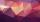Calculate quotient of geometric progression if a1=5 a a1+a2=12.
• CalculateCalculate the sum of all three-digit natural numbers divisible by five.
• This year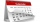This year 2020 is a leap year. How many leap years there had been be since 1904?
• Simple sequence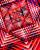Continue with this series of numbers: 1792,448, 112, _, _
• NumbersDetermine the number of all positive integers less than 4183444 if each is divisible by 29, 7, 17. What is its sum?
• Mailbox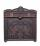Mailbox is opening at regular intervals 3 times a day. The first time is opened at 8:00 and the last at 19:00. Calculate hours when mailbox is opened during day.
• Handmade baskets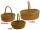Worker knits the baskets which then sells in the market. He maked seven baskets on Monday and one more each day than he did in the previous day. How many times more baskets did he make till the following Monday evening compared to the first day when he di
• Toys 3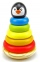Tiffany's toyshop received a shipment of 360 toys. The first day 12 were sold the second day 19 were sold and on the third day, 26 was sold. How many days will the toyshop run out of toys?
• To the cinemaJane at 8 am got message that all 1093 school pupils will go to the cinema. Within 20 min she said it to the three friends. Each of them again for 20 minutes said to the other three. In this way the message spread further. At what time all the children in

We apologize, but in this category are not a lot of examples.
Do you have an interesting mathematical word problem that you can't solve it? Submit a math problem, and we can try to solve it.

We will send a solution to your e-mail address. Solved examples are also published here. Please enter the e-mail correctly and check whether you don't have a full mailbox.

Please do not submit problems from current active competitions such as Mathematical Olympiad, correspondence seminars etc...
Division Problems. Arithmetic progression - math problems.